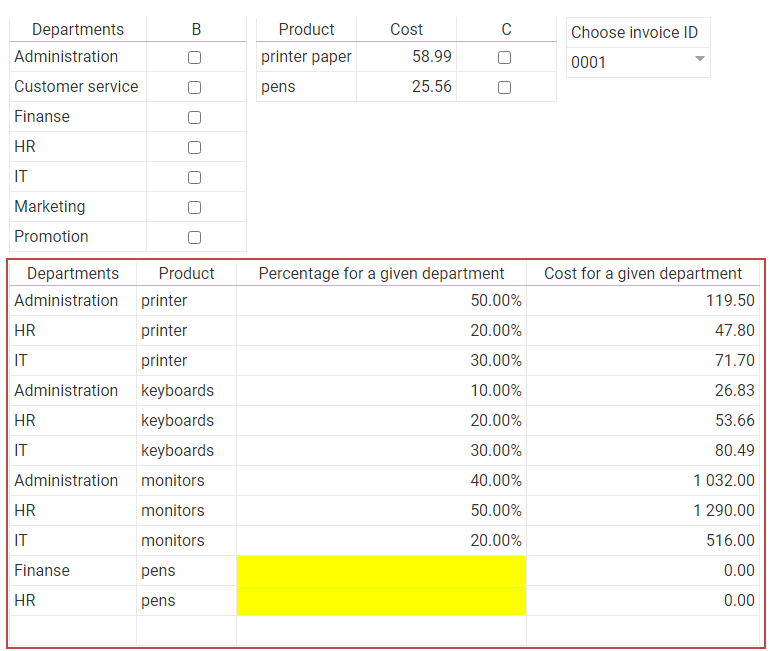# Merge tables

In this tutorial, you will learn how to use and what is the action step 'Merge Tables' on the example of a simple app for dividing expenses by departments of the company.

1. List of departments and table of invoices

1.1

Create a new list called 'COMPANY_DEPARTMENTS' with one text column, name it 'Department'. Enter different departments of the company in the rows, for example:Save the list.

1.2

Create a table called 'INVOICE_LIST' and the columns: 'ID' type: text, 'Products' type: text and 'Price' type: currency. Import the data from the bottom of the page into this table and save.

2. Source sections

2.1

You can learn how to bind sections and cells here .

Create a new app and add a table called 'Departments' in it, change the header of column A to 'Departments', and the type of column B to checkbox. Bind the table with the 'COMPANY_DEPARTMENTS' list, and A column with the 'Department' column (by dragging the table name from the data tab on the section name and dragging the 'Department' column on column A). Make column B editable.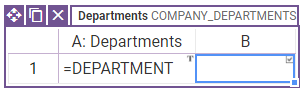2.2

Create a new two-cell spreadsheet and name it 'ID'. In the first cell, write 'Select invoice ID'. Set the second cell to editable and bind it with the 'ID' column of the 'INVOICE_LIST' table (by dragging the column name from the data tab, DO NOT drag the 'INVOICE_LIST' table name on the spreadsheet name).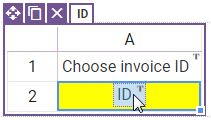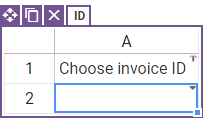2.3

Create a new table called 'Invoice_Data', name the first two columns as 'Product', 'Cost'. Change the types of the first three columns to text, currency, checkbox. Bind the table with the 'INVOICE_LIST' table, and the 'Product' and 'Cost' columns with the 'Products' and 'Price' columns. Make column C editable.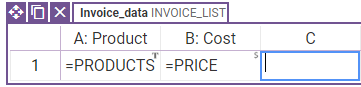In the 'Invoice_Data' table options tab, set the table visibility with the formula: '= if(isempty(ID.A2), false, true)'. In the table filter, add the condition 'ID=ID.A2' to the formula as shown below.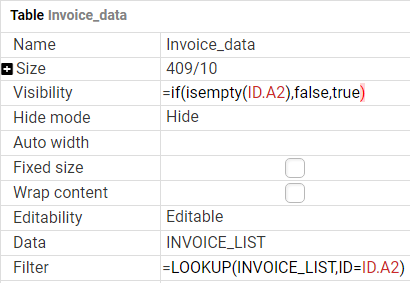Thanks to this, after selecting the invoice ID in the 'ID' spreadsheet, the 'Invoice_Data' table will appear with the products and costs of the selected invoice.

3. Target section

Create a new table called 'Dividing_costs' with 5 columns and change the column headers to: 'Departments', 'Product', 'Percentage for this department', 'Cost for this department' and 'Cost', and their types to sequentially: text, text, percentage, currency and currency. Make all cells editable except column D where you type the formula '= C*E'. Set visibility of E column to false. Allow add rows in this table.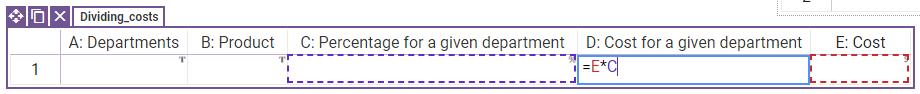It will be a table in which you will be able to determine how many percent of a given cost will be assigned to a given department.

4. Button (you can get the same effect by using the Workflow action button and creating an action from the start state to itself)

4.1

Create a button and give it a caption 'Divide the costs into departments'. Add an action Merge tables and fill action parameters as shown below.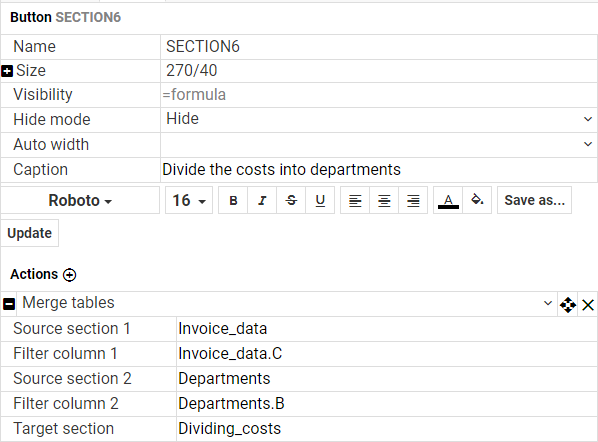Thanks to this action, selected data from the tables 'Departments' and 'Invoice_data' will appear in the 'Dividing_costs' table.

4.2

Add two more 'Reset editable cell' actions to this button. In one, as a parameter, enter the column 'Departments.B', and in the other - the column 'Invoice_Data. C'.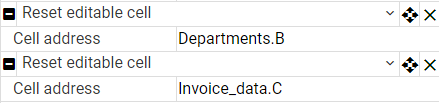This will allow you to reselect the departments and products to divide the costs.

5. Conditional formatting (optional step)

5.1

Create a spreadsheet where you will prepare three styles, save them one by one as 'Right', 'Too_much' and 'Not_enough'.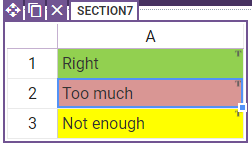5.2

In the 'Invoice_Data' table, set the visibility of column D to false, set type of this column to currency and enter the formula in it '=sumif(Dividing_costs.B,value=A,Dividing_costs.D)'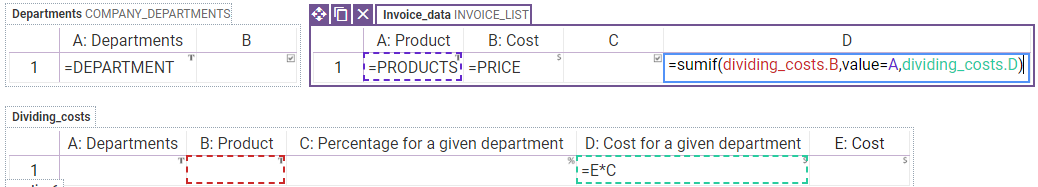The formula sumif sums the data when the given condition is true, more about SumIf. In this case, the formula selects the appropriate rows from the 'Dividing_costs' table by comparing the product name in the 'Dividing_costs' table with the 'Invoice_Data' table, and then sums up the 'Cost for a given department' for these rows.

5.3

Go back to column C of the 'Dividing_costs' table and in the column options tab, set the style to compute style using formula. In the Style formula, type the formula: =if(nth(Invoice_data.D,find(Invoice_data.A,Dividing_costs.B))=E,'Right',nth(Invoice_data.D,find(Invoice_data.A,Dividing_costs.B))>E,'Too_much','Not_enough')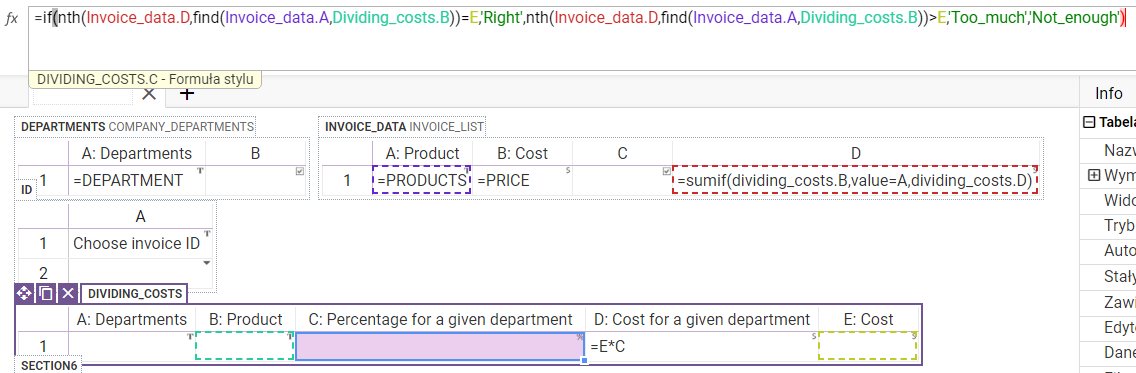Formula:

• finds the product name from column B of the 'Dividing_costs' table in column A of the 'Invoice_Data' table and gives the row number of this product using the formula find
• returns the value from column D of the table 'Invoice_Data' at the position returned by the formula find, using the formula nth
• checks if the obtained value is equal to the value of column E of the 'Dividing_costs' table, if so, the cell will be in 'Right' style; if not - checks whether the obtained value is greater than the value of column E of the 'Dividing_costs' table, if so, the cell will be in 'Too_much' style, if not the cell will be in 'Not_enough' style; it's all with the help of a function if

6. Test

6.1

Save the app and open a preview. You should see: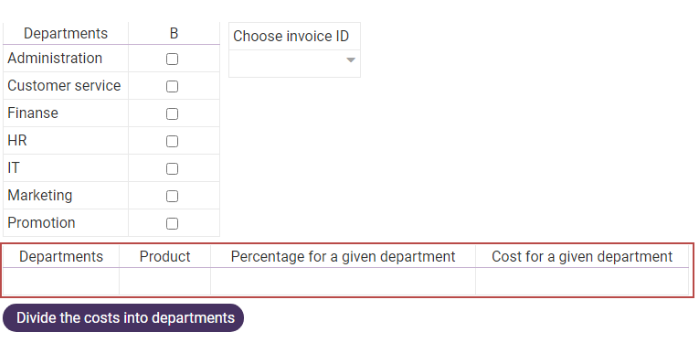6.2

Select the Invoice ID from the drop-down list. You should see a table with the products and their costs from that invoice.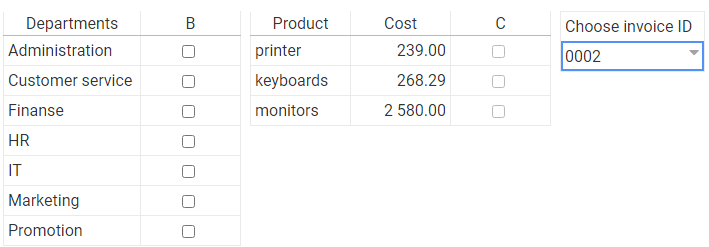6.3

Select the rows with products and costs that you want to divide and select into which departments you want to divide them by selecting the checkbox next to those rows, and then press the button. The table below should show the combined data in its rows.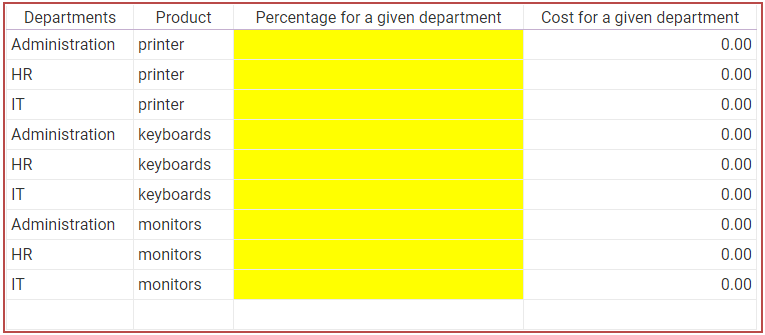6.4

Enter the percentage of shares in the given cost in the column. Do it correctly at first, then make a deliberate mistake in entering percentages as shown below. Cells should properly change color when it is right, too much, and too little.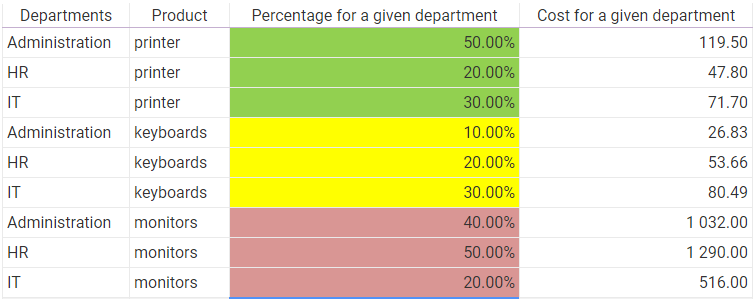6.5

You can also select a different invoice, select products and departments and press the button. New rows with the appropriate data should appear.